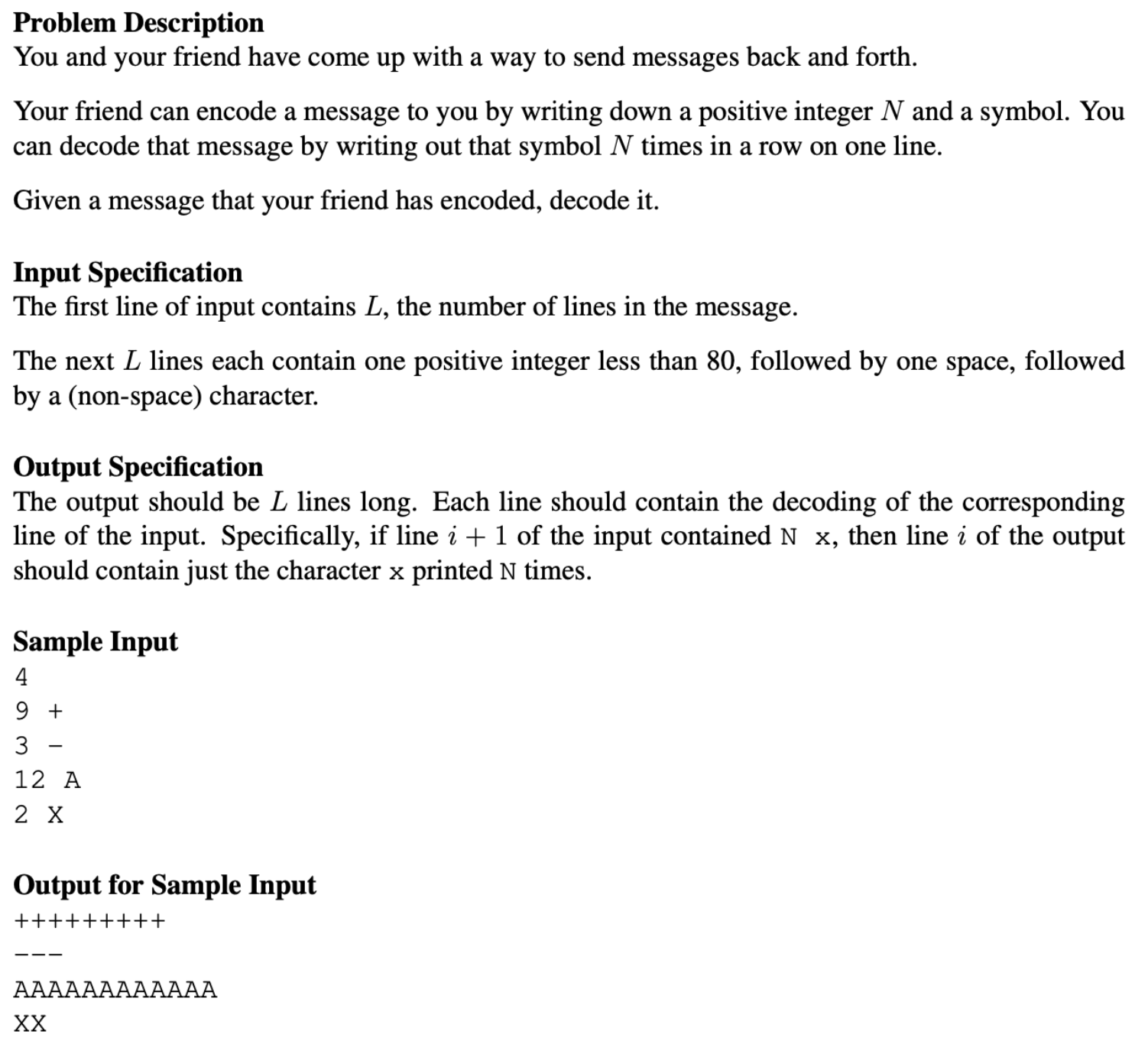# Java unable to recognise special character after space

I have to write a program for the following problem:I have written the following code:

``````package secret_message;
import java.util.Scanner;
public class Secret_Message {
public static void main(String[] args) {
Scanner sc = new Scanner (System.in);
int lines, i, codenumber, k;
String code, codepart, codechar2;
char codecharacter1, codecharacter2;
lines = sc.nextInt();
codepart = "";
for (i = 1; i<=lines; i++)
{
code = sc.next();
codecharacter1 = code.charAt(0);
codecharacter2 = code.charAt(1);
codenumber = Character.getNumericValue(codecharacter1);
for (k=1; k<=codenumber; k++)
{
codechar2 = Character.toString(codecharacter2);
codepart = codepart + " " + codechar2;
}
System.out.println(codepart);
codepart = "";
}
}
}
``````

It works perfectly when my input on each line does not have a space (for example if I write "9+" instead of "9 +"). However, on writing "9 +" for example, I get the following error: "String index out of range". I tried changing `codecharacter2 = code.charAt(1);` in my code to `codecharacter2 = code.charAt(2);` and `codecharacter2 = code.charAt(3);` with no success. I also attempted to create a new variable using `code.replaceAll("\s", "");` but this would also remove the "+" in "9 +".

I know it only makes a subtle difference to include the space but the question explicitly states the need for it. Hence, I would appreciate any help on how I can get around this problem!

## Solution

The problem is here `code = sc.next();` this means you are only taking the first token from the input which is the first character. Instead you need to take the entire line as per your logic i.e.,

``````code = sc.nextLine();
``````

Better solution would be to take the integer and character separately. Check below code:

``````    for (i = 1; i<=lines; i++)
{
codenumber= sc.nextInt();
codechar2 = sc.nextLine().charAt(0);
for (k=1; k<=codenumber; k++)
{
codepart = codepart + " " + codechar2;
}
System.out.println(codepart);
codepart = "";
}
``````﻿﻿